# Recursion facts for kids

Kids Encyclopedia Facts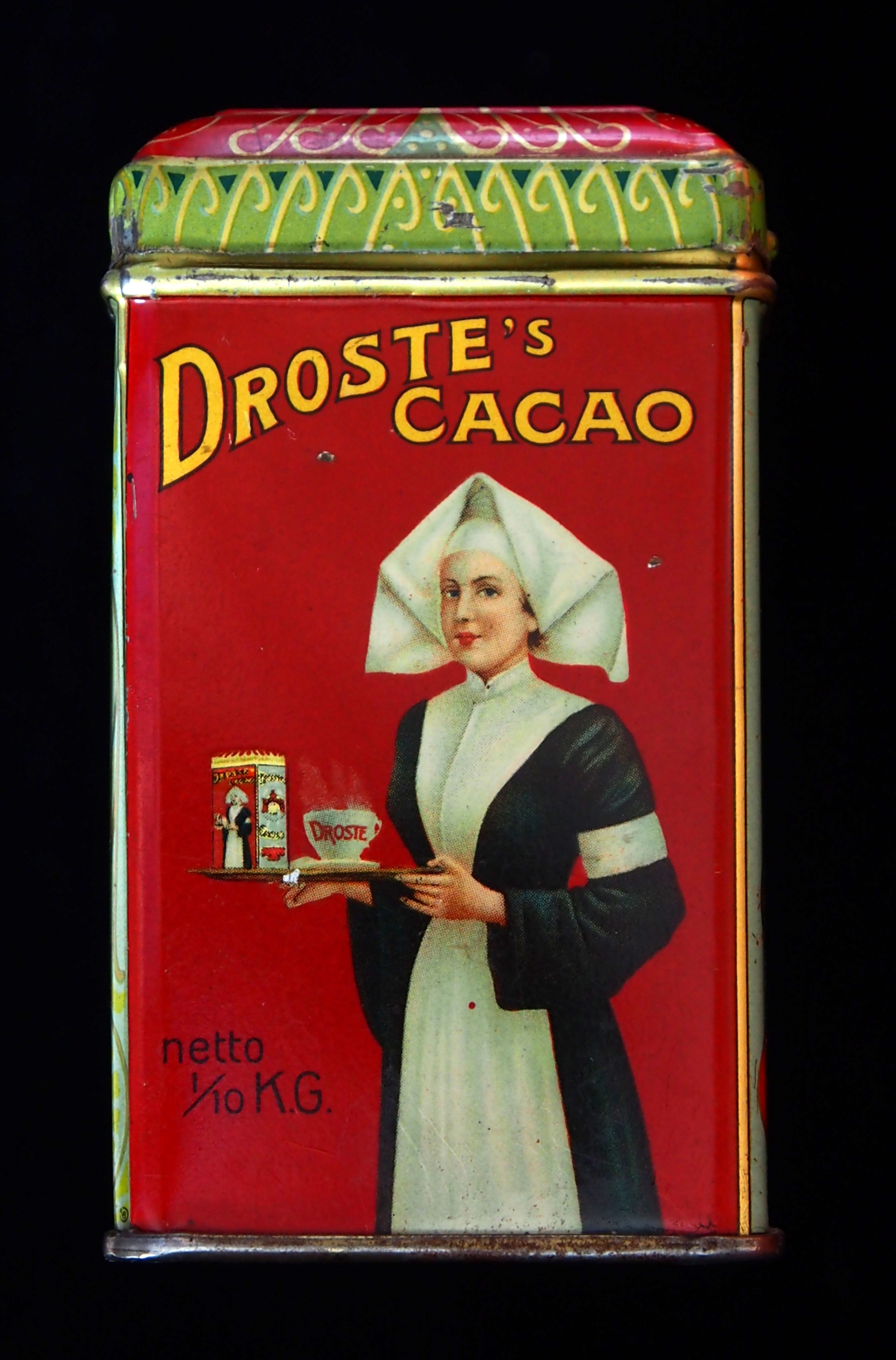A visual form of recursion is the Droste effect. It leads to self-similar images.

Recursion is a word from mathematics and computer science. It is used to define a thing, such as a function or a set. A recursive definition uses the thing it is defining as part of the definition.

## Description

Usually, a recursive function refers to itself in some cases (or inputs), but not in every case. A function that referred to itself in every case would never terminate.

When a function refers to itself, it often uses a smaller input than the input it was given. In this way, it can solve a problem by first solving a simpler version of the problem.

## Example

An example of a recursive function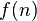$f(n)$ is:

• If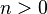$n > 0$ then return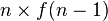$n \times f(n-1)$.
• If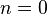$n = 0$ then return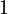$1$.

This function computes the factorial of a natural number. It works because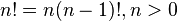$n!=n(n-1)!, n > 0$ and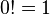$0!=1$.

The definition has two cases: a recursive case for$n>0$, and a case for$n=0$ that is not recursive. The case that is not recursive is called a "base case".

## Uses

Recursion can be used to write computer programs. A program that uses recursion may be easier to write and understand than a program that does the same thing without recursion.

Recursion is used in mathematics to prove theorems. This method is called induction.

The idea of recursion can be seen in art and language. For example:

• A fractal image contains smaller versions of itself.
• In the rules of grammar, a sentence can be part of another sentence.

## Images for kidsRecursion Facts for Kids. Kiddle Encyclopedia.